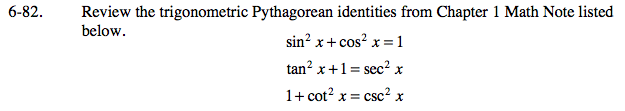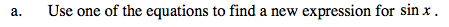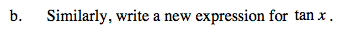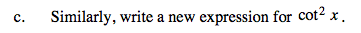### Home > CALC > Chapter 6 > Lesson 6.3.1 > Problem6-82

6-82.
1. Review the trigonometric Pythagorean identities from Chapter 1 Math Note listed below. Homework Help ✎

sin2x + cos2x = 1
tan2x + 1 = sec2x
1 + cot2x = csc2x

1. Use one of the equations to find a new expression for sin x.

2. Similarly, write a new expression for tan x.

3. Similarly, write a new expression for cot2 x.sin2x = 1 − cos2xRefer to part (a).Refer to part (a).